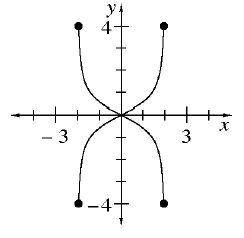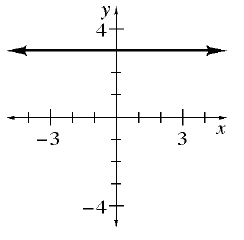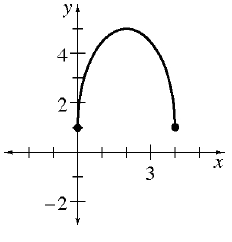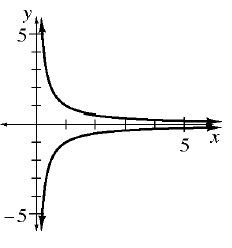### Home > AC > Chapter 11 > Lesson 11.3.1 > Problem11-100

11-100.

For each relation graphed in problem 11-99, explain whether it is a function. If the relation is not a function, give a reason to support your claim.

A function can have only one output value $\left(y\right)$ for each input value $\left(x\right)$.

1.It is not a function because there are two $y$-values for at least one $x$-value.

1.It is a function.

1.It is a function.

1.It is not a function because there are two $y$-values for at least one $x$-value.